OPTIMAL ALLOCATION OF EFFORT IN PACKET SWITCHING NETWORKS WITH GENERALLY DISTRIBUTED TRANSMISSION TIMES

by

Phil Pollett
Department of Mathematics
The University of Queensland

PACKET SWITCHING NETWORKS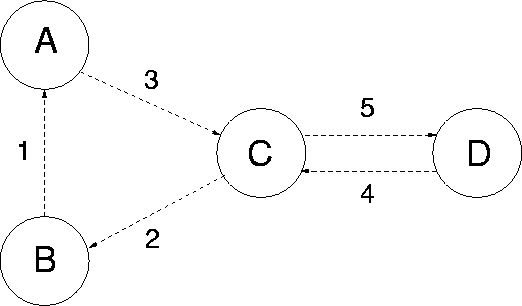A packet switching network with 4 nodes (labelled A,B,C and D) and 5 links (labelled 1,2,..5)

PACKET SWITCHING NETWORKS

N switching nodes (labelled)

J links (labelled)

Poisson traffic on route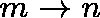at rate(type-mn traffic)

Common expected message length:(bits)

(Message lengths have an arbitrary distribution which does not depend on type)

Transmission rate on link j is(bits/sec.)

(There is a first-come first-served (FCFS) discipline at each link)

ROUTING MECHANISMS

Fixed routing

Define R(m,n) to be the collection of (distinct) links used by type-mn traffic: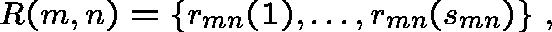whereis the number of links on routeandis the link used at stage s.

Random alternative routing

This can be accommodated within the framework of fixed routing by allowing a finer classification of type (mni):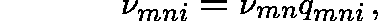whereis the probability that alternative route i is chosen; there is fixed set of alternative routes for each OD pair (m,n).

PACKET SWITCHING NETWORK AND CORRESPONDING QUEUEING NETWORK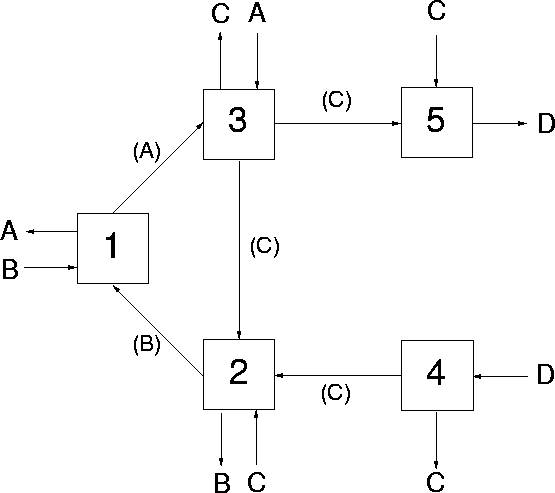A NETWORK OF QUEUES

Linksqueues

Messagescustomers

T - set of customer types- arrival rate of type-t customers

(Independent Poisson streams)

Route for type-t customers:A NETWORK OF QUEUES

If message lengths have an exponential distribution the links behave independently (indeed, as if they were isolated), each with independent streams of Poisson offered traffic (independent among types). For example, ifso that the arrival rate at link j is given byand the demand by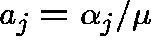(bits/sec), then, if the system is stable (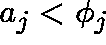for each j), the expected number of messages at link j isand the expected delay isTHE INDEPENDENCE ASSUMPTION

Kleinrock (1964) proposed the following assumption: that successive messages requesting transmission along any given link have lengths which are independent and identically distributed, and that message lengths at different links are independent.

Thus, we shall assume that at link j message lengths have a distribution function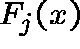which has meanand variance.

Even under this assumption, the model (now a network ofqueues with a FCFS discipline) is not analytically tractable. To make progress, we shall use the Residual-life Approximation (Pollett (1984)).

THE RESIDUAL LIFE APPROXIMATION

Letbe the distribution function of the queueing time at link j: the time a message spends in the buffer before transmission. The Residual-Life Approximation (RLA) provides an accurate approximation for:whereanddenotes the n-fold convolution of. The distribution of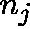, the number of messages at link j, used in (2) is that of the corresponding quasireversible network of symmetric queues obtained by imposing a symmetry condition at each link j. In the present context, this amounts to replacing FCFS by a last-come first-served (LCFS) discipline.

THE RESIDUAL LIFE APPROXIMATION

One immediate consequence of (2) is that the expected queueing time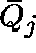is approximatelywhereis the expected number of messages at link j in the quasireversible network. Hence, the expected delay at link j is approximated as follows:In the RLA, it is onlywhich changes when the service discipline is altered. For the present FCFS disciplineis given byOPTIMAL ALLOCATION OF EFFORT

We shall minimize the average network delay, or equivalently the average number of messages in the network:(using the RLA for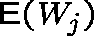).

\$F - overall network budget(\$-seconds/bit) - cost of operating link j

(The cost of operating link j is proportional to the capacity)

Thus, we should choose the capacities subject to the cost constraintTHE PROBLEM

Letbe the squared coefficient of variation ofand let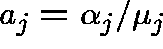.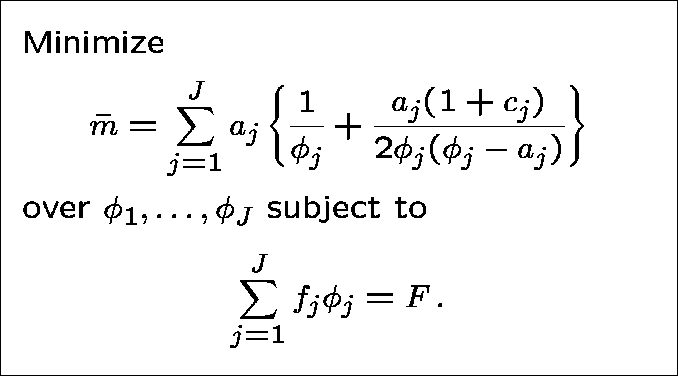Introduce a lagrange multiplier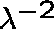; our problem then becomes one of minimizingSetting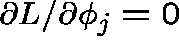yields a quartic polynomial equation in:where.

Find solutions such that(recall that this latter condition is a requirement for stability).

Using the transformation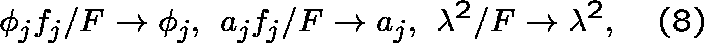the problem reduces to one with unit costs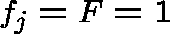; equation (6) becomesand the constraint becomesEXPONENTIAL SERVICE TIMES

If transmission times are exponentially distributed (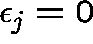for each j) it is easy to verify that (10) has a unique solution ongiven by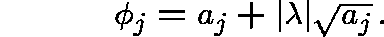Upon application of the constraint (12) we arrive at the optimal capacity assignmentfor unit costs. In the case of general costs this becomesafter applying the transformation (8). This is a result obtained by Kleinrock (1964).

THE GENERAL CASE

We shall adopt a perturbation approach, assuming that the lagrange multiplier and the optimal allocation take the following forms:where by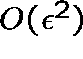we mean terms of order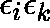. The zero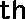order terms come from Kleinrock's solution:whereFIRST-ORDER SOLUTION

On substituting (14) into (10) we obtain an expression for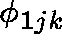in terms of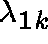, which in turn is calculated using the constraint (12) and by setting(the Kronecker delta).

To first order, the optimal allocation iswhereSENSITIVITY

Let,, be the new optimal allocation obtained after incrementing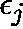by a small quantity. We find that, to first order in,and, for,